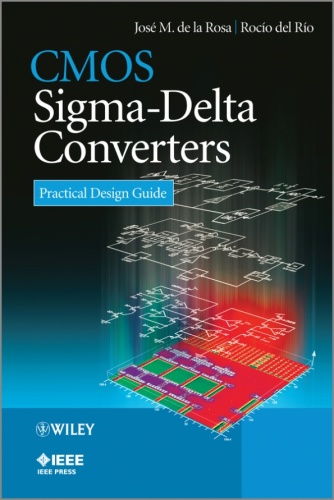CMOS Sigma Delta Converters  Practical Design Guide (EPUB)
Type : epub |
Size : 39.81 MB |
English |

Descirption: A comprehensive overview of Sigma-Delta Analog-to-Digital Converters (ADCs) and a practical guide to their design in nano-scale CMOS for optimal performance.
This book presents a systematic and comprehensive compilation of sigma-delta converter operating principles, the new advances in architectures and circuits, design methodologies and practical considerations - going from system-level specifications to silicon integration, packaging and measurements, with emphasis on nanometer CMOS implementation. The book emphasizes practical design issues - from high-level behavioural modelling in MATLAB/SIMULINK, to circuit-level implementation in Cadence Design FrameWork II. As well as being a comprehensive reference to the theory, the book is also unique in that it gives special importance on practical issues, giving a detailed description of the different steps that constitute the whole design flow of sigma-delta ADCs.
The book begins with an introductory survey of sigma-delta modulators, their fundamentals architectures and synthesis methods covered in Chapter 1. In Chapter 2, the effect of main circuit error mechanisms is analysed, providing the necessary understanding of the main practical issues affecting the performance of sigma-delta modulators. The knowledge derived from the first two chapters is presented in the book as an essential part of the systematic top-down/bottom-up synthesis methodology of sigma-delta modulators described in Chapter 3, where a time-domain behavioural simulator named SIMSIDES is described and applied to the high-level design and verification of sigma-delta ADCs. Chapter 4 moves farther down from system-level to the circuit and physical level, providing a number of design recommendations and practical recipes to complete the design flow of sigma-delta modulators. To conclude the book, Chapter 5 gives an overview of the state-of-the-art sigma-delta ADCs, which are exhaustively analysed in order to extract practical design guidelines and to identify the incoming trends, design challenges as well as practical solutions proposed by cutting-edge designs.
Offers a complete survey of sigma-delta modulator architectures from fundamentals to state-of-the art topologies, considering both switched-capacitor and continuous-time circuit implementations
Gives a systematic analysis and practical design guide of sigma-delta modulators, from a top-down/bottom-up perspective, including mathematical models and analytical procedures, behavioural modeling in MATLAB/SIMULINK, macromodeling, and circuit-level implementation in Cadence Design FrameWork II, chip prototyping, and experimental characterization.
Systematic compilation of cutting-edge sigma-delta modulators
Complete description of SIMSIDES, a time-domain behavioural simulator implemented in MATLAB/SIMULINK
Plenty of examples, case studies, and simulation test benches, covering the different stages of the design flow of sigma-delta modulators
A number of electronic resources, including SIMSIDES, the statistical data used in the state-of-the-art survey, as well as many design examples and test benches are hosted on a companion website
Essential reading for Researchers and electronics engineering practitioners interested in the design of high-performance data converters integrated in nanometer CMOS technologies; mixed-signal designers.

Clic Down
From Here
From Here

https://nitroflare.com/view/CFDFEC571D161A9/...9781118568439.epub

From Here